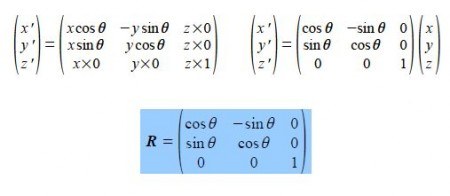# Molecular Symmetry Operations, Linear Transformations

The positions of atoms in a molecule can be defined in terms of Cartesian coordinates x, y, z. In the analysis of molecular geometry there is often a need to calculate atomic coordinates and then subsequently find their new positions (using matrices) due to the symmetry operations of reflection, inversion, rotation and rotation-reflection.

Consider the anticlockwise rotation, around the z-axis, of an atom with coordinates X(x, y) through an angle 0 to the new position X ‘(x’, y’) as in below Figure,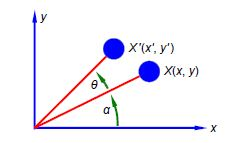What is the appropriate 2×2 matrix R for this rotational transformation such that,

X ‘ = XR

Assuming that the z-axis is pointing out from the xy-plane (the right hand rule), how would matrix R need to be modified for a more general anticlockwise rotation about the z-axis of a point with coordinates (x, y, z)?

Solution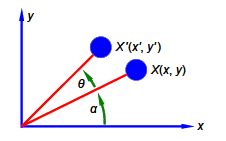If the distance from the origin is r then for X and X’ we have,

X (x = r cos α    y = rsinα )   X ‘ (x’ = rcos (α +θ)     y’ = rsin (α +θ))

Expanding the double angles using the trig formula cos (A + B) = (cosA cosB) — (sinA sinB) and the formula for sin (A + B) = (sinA cosB) + (cosA sinB). For X’ we have,

x’ = r(cos α cosθ — sinα sin θ)   y’ = r(sin α cosθ  + cos α sinθ )

Noting that the change from (x, y) to (x’, y) only involves the value of 0 (as r and a are constants) we can put the change due to the rotation, i.e. the transformation, into a matrix form,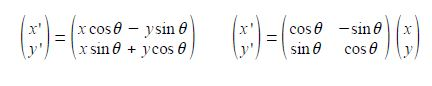The rotation matrix around the z-axis R is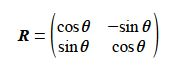Extending the rotation matrix R to the more general anticlockwise rotation about the z-axis of a point with coordinates (x, y, z) in Molecular Symmetry Operations.